﻿ Potential flow in backstep geometry - XSim

# Potential flow in backstep geometry

Update: June 1, 2017
OpenFOAM 4.x

$FOAM_TUTORIALS/basic/potentialFoam/pitzDaily ## Summary Potential flow in backstep geometry (perfect fluid flow without vortices)will be solved The fluid flows in from the inlet region with velocity (10, 0, 0) and out from the outlet region. Set the no-slip condition for the upper and lower surfaces in the Y direction, and the calculation is performed as a two-dimensional problem with a single mesh in the Z direction.Model geometry The meshes are as follows.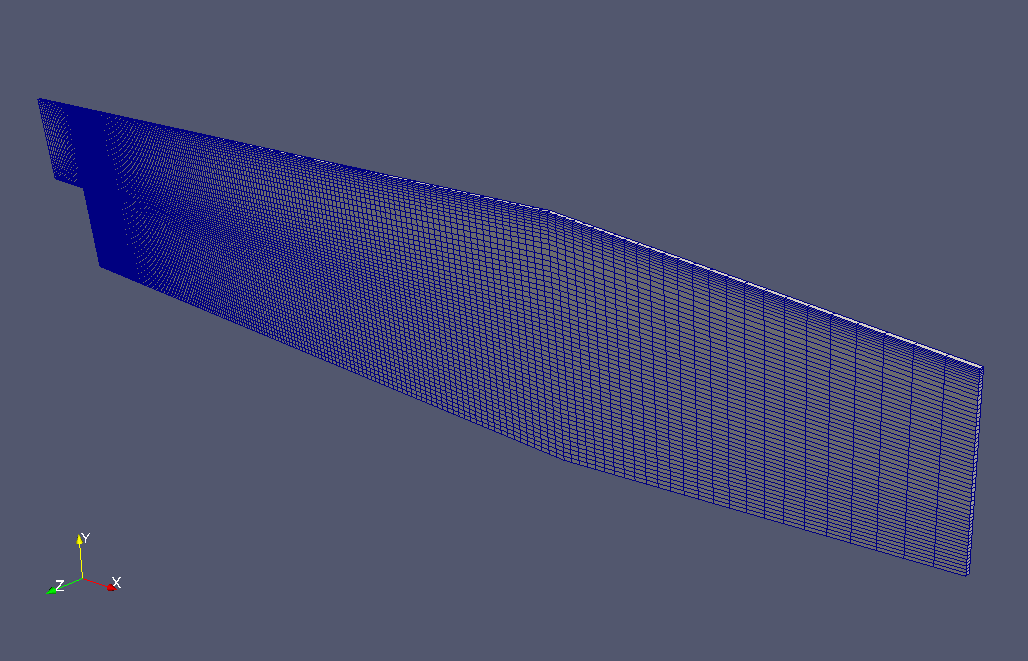Meshes After the potential flow calculation, the flow function is calculated and output. To visualize the stream function, check the "streamFunction" checkbox in the "Properties" tab on ParaView.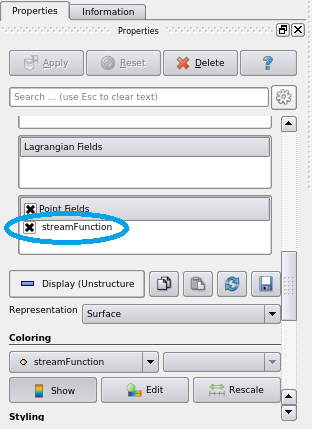Check the "streamFunction" The calculation result is as follows.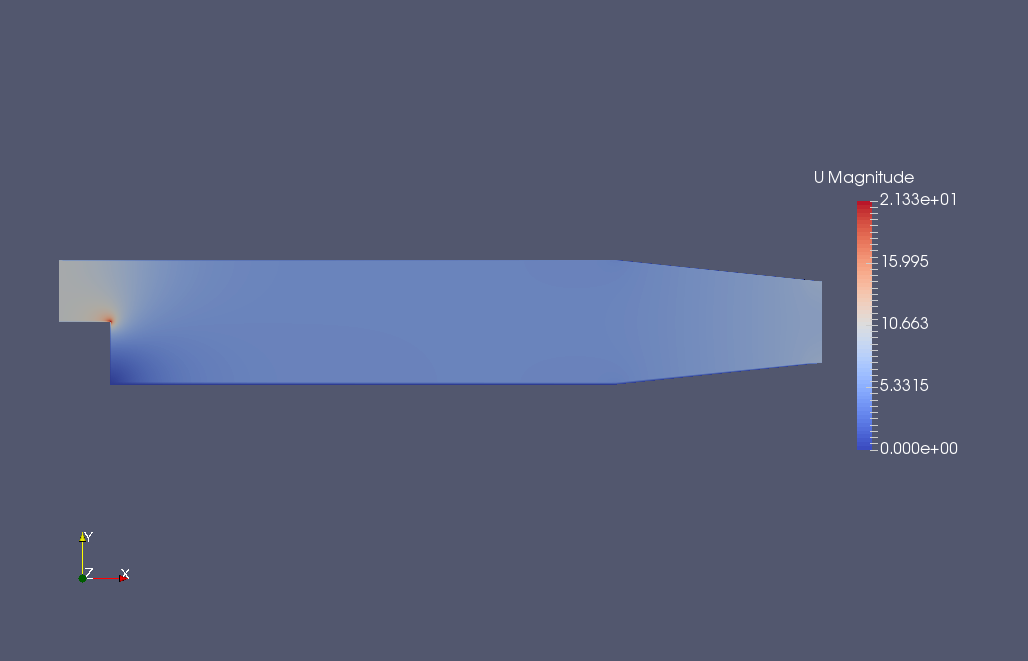Flow velocity (U)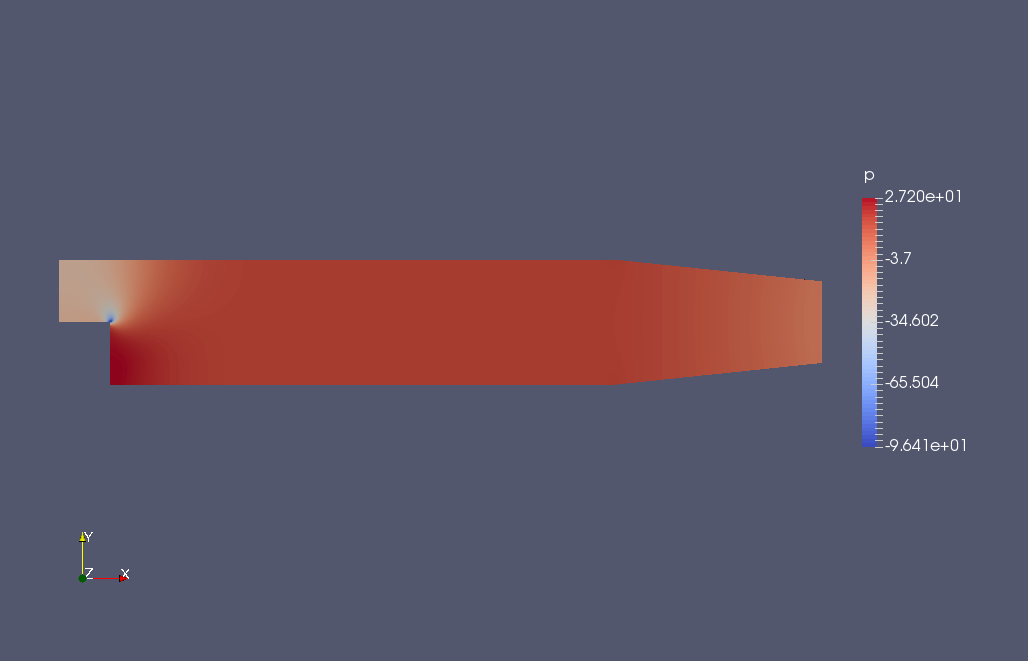Pressure (p)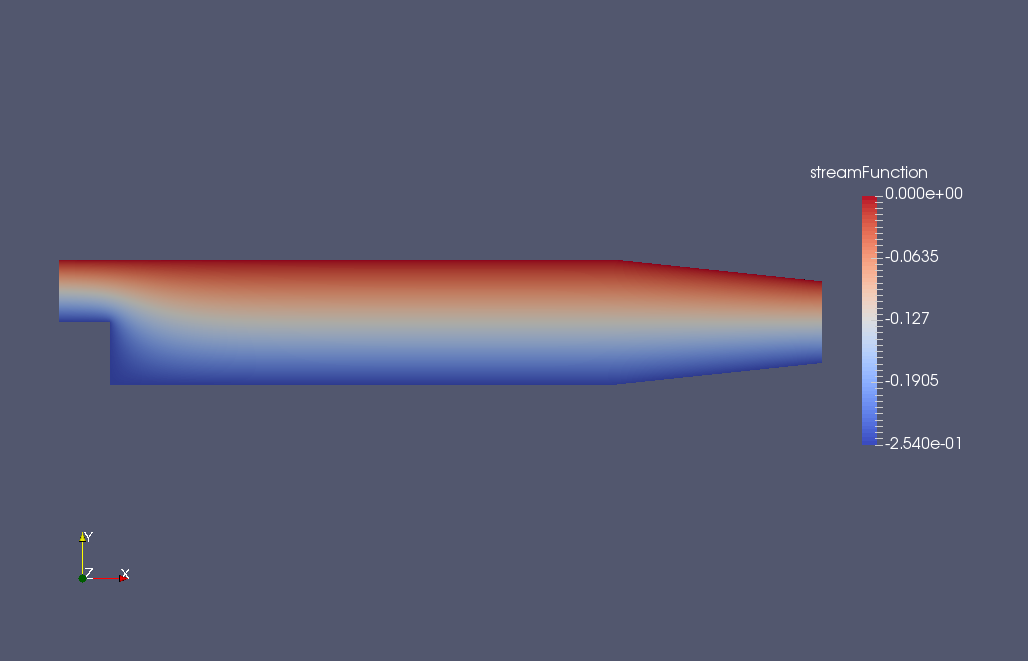Stream function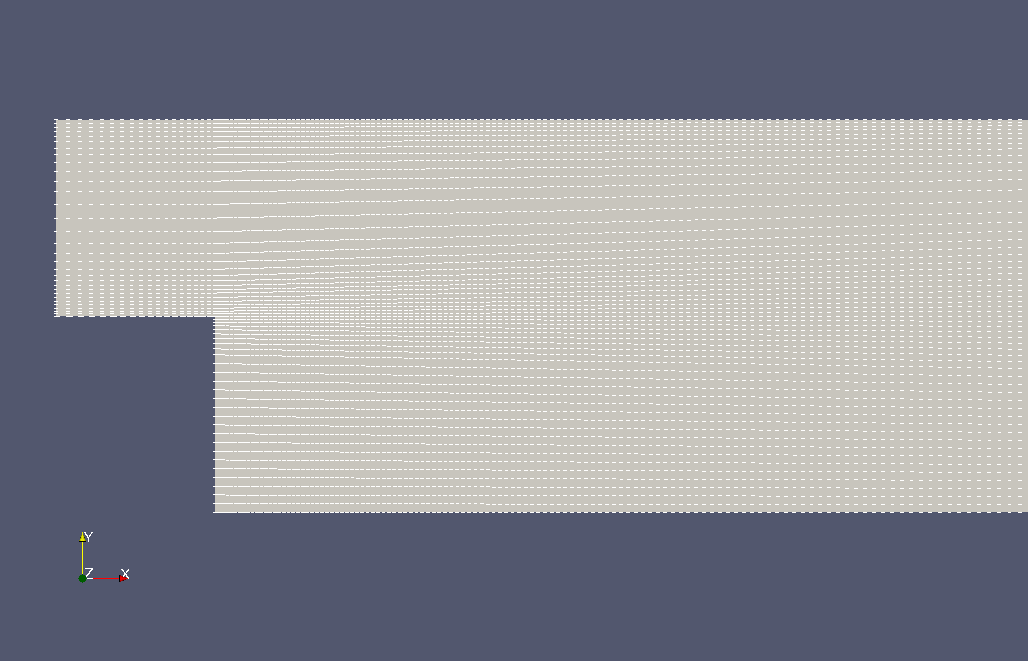Flow vector around the step Since it is a potential flow, no vortex is generated. ## Commands cp -r$FOAM_TUTORIALS/basic/potentialFoam/pitzDaily pitzDaily
cd pitzDaily

cp -r 0.orig 0
blockMesh
potentialFoam -writePhi -writep
streamFunction

paraFoam

The boundary conditions are in the 0.orig directory, and the calculation results are overwritten in the 0 directory.

The solver arguments specify the output of the flow potential field (-writePhi), and output of the pressure field (-writep).

After the calculation, the utility streamFunction is used to calculate and output the stream function.

## Calculation time

--- *Single, Core(TM) i7-2600 CPU @ 3.40GHz 3.40GHz